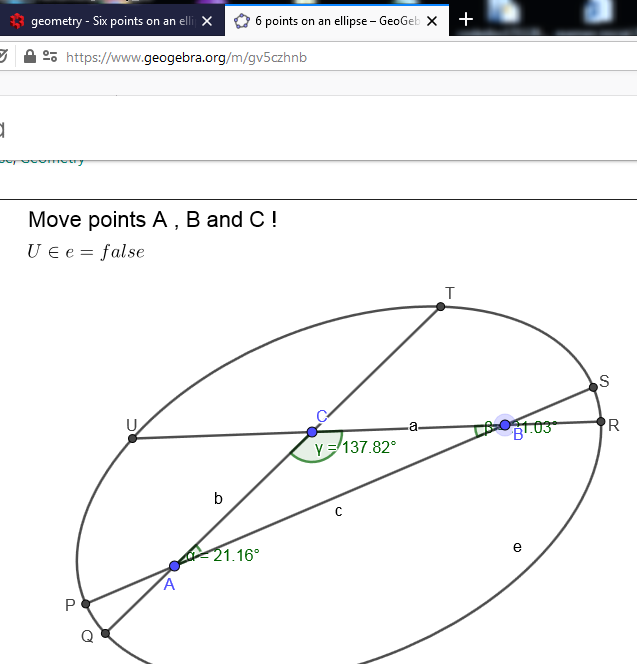# GeoGebra ∈ gives an incorrect result?

PrimusLab shared this question 11 months ago

I made a Geogebra applet which tests if the point lies on the specific conic section . I have used simple test : U ∈ e where U is the point and e is the conic section. However, applet's results and the proof given in this answer do not match. In other words my applet gives "true" as an answer although it should be "false". Is this the known issue?2

U ∈ e means is U inside e ?2

"U ∈ e" is true if U is on the border or inside the ellipse (Note: e has an area)

I assume you mean:

Distance(U,e)<=10^-14 (with consideration of binary rounding errors)2